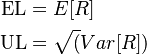# Difference between revisions of "Expected Loss versus Unexpected Loss"

## Expected Loss versus Unexpected Loss

Expected Loss versus Unexpected Loss highlights a very general (and sometimes confusing) strategy in Quantitative Risk Management to decompose estimates of potential future Loss into an expected component (EL, Expected Loss)) and an uncertain element (UL, Unexpected Loss).

In the simplest case where risk is represented by a Random Variable, the decomposition has an natural mathematical correspondence:\begin{align} \mbox{EL} & = E[R] \\ \mbox{UL} & = \sqrt(Var[R]) \end{align}

## Issues and Challenges

A number of confusions may emerge from informal or ambiguous use of language

• The 'expectation' in Expected Loss does not imply a certain outcome
• The expectation in Expected Loss does not mean the most likely outcome but the scenario average
• The unexpected in Unexpected Loss can be captured by many different measures (e.g, quantile or other tail Risk Measure)

---